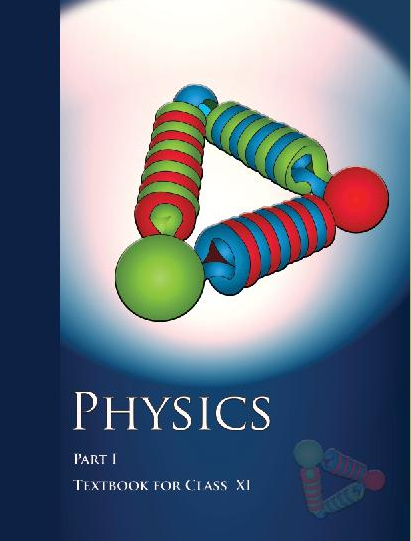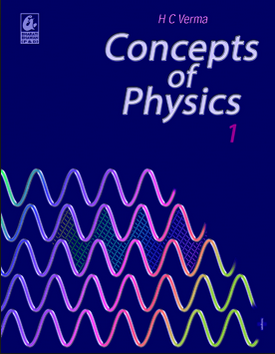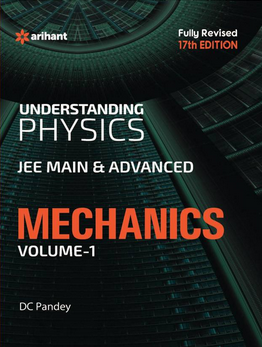# Laws of motion (Weightage 3%)   Share

### Topics from Laws of motion

• Force and Inertia,Newton's First Law of motion (15 concepts)
• Momentum,Impulse (4 concepts)
• Newton's Second Law of motion;Newton's Third Law of motion. (2 concepts)
• Law of conservation of linear momentum and its applications (4 concepts)
• Equilibrium of concurrent forces. (26 concepts)
• Static and Kinetic friction, laws of friction, rolling friction. (32 concepts)
• Dynamics of uniform circular motion: Centripetal force and its applications. (27 concepts)
• Friction (20 concepts)
• Newtons First Law (5 concepts)
• Newtons 2nd and 3rd Laws of motion (2 concepts)
• Applications of Newtons 2nd and 3rd Laws of motion (11 concepts)
• Dynamics of uniform circular motion (8 concepts)

### Important Books for Laws of motion

•••Exams
Articles
Questions